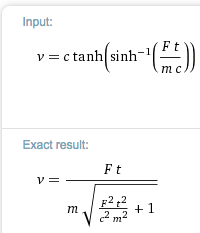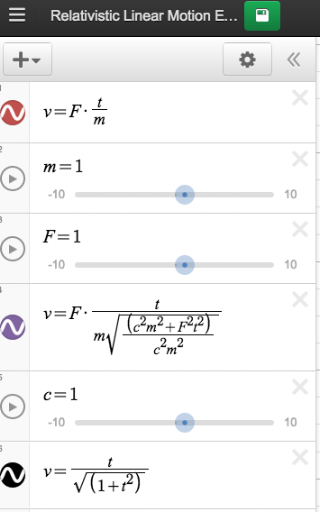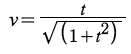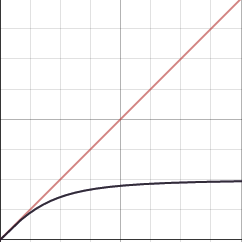## Sunday, October 19, 2014

### The relativistic version of the linear motion equation: velocity = acceleration*time.

My son is starting to do … physics.

It is fair to say I am more excited about this than he is. There is a reason I have a copy of the 1973 Edition of Misner, Thorne and Wheeler’s Gravitation (\$35!) on my bookcase. I’m saving it for retirement, by which time Desmos.com will breeze through all the visualizations. It is true I was a failure (non-physicist) at Caltech, but there’s still time…

Ahem. So when #w was given an assignment to create a poster about linear motion, with equations on time, velocity, constant acceleration and the like, I thought it would be fun to show that the equations of linear motion are merely a low velocity approximation to the relativistic equations of linear motion. I was sure a quick Google search would turn up a simple simulation that would look like:

v=a*t (starting at rest velocity after time t is acceleration times t)

Riiiight. I’m sure that simulation exists, but I was never able to find it. With a bit of thought about the problem I realized I might be better off dropping the tricksy concept of acceleration and looking for a relativistic version of:

v= (F/m)*t (since change in velocity is Force*Time/Mass. Push a trike, push a truck, which moves faster?)

That found an “off-topic”  stack overflow article which was just a few parentheses short of the good-enough equation : v = c * tanh(asinh((F*t)/(m*c))).

This is the first time I’ve personally run into hyperbolic trig functions, so I thought that was pretty cool, especially since I’d just read Jon Butterworth’s lovely description of how one hops from simple physics to quantum physics simply by tossing the square root of -1 (i) into a classical wave equation (uses Euler’s Formula, so extra points). That article used the familiar sin/cos functions, so I was getting an extra dose of trig.

Plugging v = c * tanh(asinh((F*t)/(m*c))) into Wolfram Alpha gave me this cool output (yes, the AIs wll be our death, but for now they’re fun):Now that is what I was looking for! It clearly reduces to v = (F/m)*t when the squared stuff is relatively small. 

We can now compare my son’s high school textbook linear motion equation (v=F/m*t) to the relativistic equation, using the sneaky physics trick of geometrized units so c=1. Just to make things even nicer I’ll arbitrarily set the applied force to “1” (some unit) and the mass to “1” (some unit). This is what Desmos shows:The last shows how simple this is in geometrized units (v is change in velocity over time t in our funky units):and here’s the magic graph that shows what happens as velocity (y axis) approaches “1” (speed of light) in the original linear motion equation and the relativistic version:Yeah, hits the speed limit. 

 The iron law of StackOverflow is that any article of interest will sooner or later by marked off-topic.

 I discovered the problem when I plugged it into Wolfram alpha, I had to do some web searches and play around the parens to get the correct expression.

 Incidentally, if you’d told me in 1995 that we would still lack easy entry of mathematical notation into web pages in 2014 I’d have assumed some kind of worldwide civilizational collapse.

 Once I had this expression, which was provided by the Wolfram AI, I found a discussion thread (scroll down) telling me it can be derived by “substituting F/m for a in the Baez equations(where m is the proper mass) and dividing by c where the tanh/sinh stuff is related to “proper and coordinate time”.

 I’ve done great violence here to the principles of general relativity — the meaning of time and distance are entirely dependent on frames of reference and I’ve glossed over all of that — largely because, you know, I’m not a physicist. It is kind of neat, however, what one can kludge together with a handful of web tools. In the general vein of non-physicist at play, it’s fun to compare this to Butterworth’s masterly representation of quantum physics using high school math. I again am left with feeling that physics will be easier to understand once we figure out what “distance” and “time” emerge from.